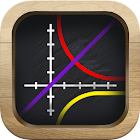Graph Lite

All Android applications categories

All Android games categories# Graph Lite

341 6

6 Users
rating

## Screenshots

Description

This graphing calculator program supports more than a dozen different functions from trigonometry, calculus, and algebra. If you like to see things when learning them and enjoy playing around with different variations of new concepts then this free program is for you. Math students worldwide benefit from the 3D Graphs.

You can graph lines, parabolas, hyperbolas, sine or cosine waves, tangent, logarithms, even exponentials. The math ranges from Algebra 1 through Algebra 2, Trigonometry, and even Pre-Calculus. With this App you can graph most Calculus equations simply using the custom math keyboard.

By using OpenGL advanced 3D graphics technology this app achieves unparalleled scrolling and zooming speed and smoothness. We use standard pinch-zoom gestures to allow you to get started without delay. Whether you are a math student, teacher, or just an enthusiast, we think you'll agree that beautiful plots are easy to create for fee.

Playing with or practicing with this program can help you to understand mathematical equations at a deeper level using visual intuition. This is a great way to prepare for standardized tests or ensure that you will be able to work at high speed for AP Mathematics exams. The bright colors and smooth shading create a memorable experience for each equation plot. If you like math, you will enjoy this app.

Tags: math graph , math graphs , 3d graph , graph , math graphing , graphe maths , graph 3d , different math graph , eval graph 3d gratis

from 341 reviews

"Good"

6